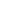# Free CBSE Class 10 Mathematics UNIT V: Trigonometry Some Applications of Trigonometry Worksheets

Download free printable Some Applications of Trigonometry Worksheets to practice. With thousands of questions available, you can generate as many Some Applications of Trigonometry Worksheets as you want.

## Sample CBSE Class 10 Mathematics UNIT V: Trigonometry Some Applications of Trigonometry Worksheet Questions

1.

tan2A=?

1.

2tanA/1+tan^2A

2.

2tanA/1-tan^2A

3.

2tanA/1+tan2A

4.

2tanA/1-tan2A

2.

If sin A – cos A = 0, then the value of sin4 A + cos4 A is

1.

1

2.

2

3.

3/4

4.

1/2

3.

If in ΔABC, ∠C = 90°, then sin (A + B) =

1.

0

2.

1/2

3.

1/√2

4.

1

4.

Given that sin θ = a/b, then tan θ =

1.

b/√(b2-a2)

2.

√(b2-a2)/b

3.

a/√(b2-a2)

4.

√(b2-a2)/a

5.

What is the maximum value of 1/cosecA ?

1.

0

2.

1

3.

1/2

4.

2

6.

What is the minimum value of sin A, 0 ≤ A ≤ 90°

1.

1

2.

0

3.

-1

4.

1/2

7.

If sec A + tan A = x, then sec A =

1.

(x2-1)/x

2.

(x2-1)/2x

3.

(x2+1)/x

4.

(x2+1)/2x

8.

If y sin 45° cos 45° = tan2 45° – cos2 30°, then y =

1.

-1/2

2.

1/2

3.

-2

4.

2

9.

sin2B = 2sinB is true when B is equal to

1.

90°

2.

60°

3.

30°

4.

10.

If   x tan 45° sin 30° = cos 30° tan 30°    , then x is equal to

1.

1/2

2.

1/√2

3.

√3

4.

1

Worksheets by UrbanPro

Our worksheets are designed to help students explore various topics, practice skills and enrich their subject knowledge, to improve their academic performance. Designed by Experts who have extensive experience and expertise in teaching a subject, these worksheets will improve your child's problem-solving skills and subject knowledge in a fun and interactive manner.
Check out our free customized worksheets across school boards, grades, subjects and levels of subject knowledge. You can download, print and share these worksheets with anyone, anywhere, anytime!

Get a custom worksheet to practice!

Select your topic & see the magic.

subjectSelect Chapter(s)

Chapters & Subtopics